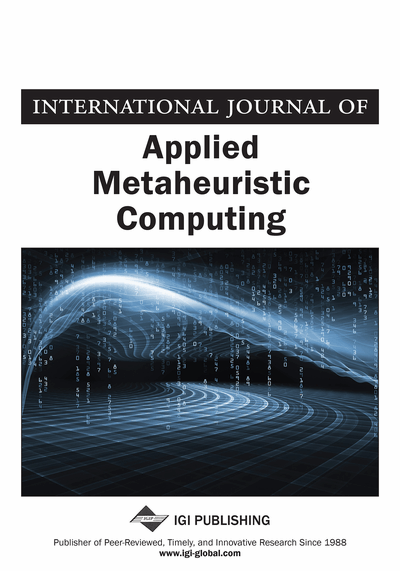# Metaheuristic Search with Inequalities and Target Objectives for Mixed Binary Optimization – Part II: Exploiting Reaction and Resistance

Fred Glover (OptTek Systems, Inc., USA) and Saïd Hanafi (University of Lille -Nord de France, UVHC and LAMIH, France)
DOI: 10.4018/jamc.2010040101

## Abstract

Recent metaheuristics for mixed integer programming have included proposals for introducing inequalities and target objectives to guide this search. These guidance approaches are useful in intensification and diversification strategies related to fixing subsets of variables at particular values. The authors’ preceding Part I study demonstrated how to improve such approaches by new inequalities that dominate those previously proposed. In Part II, the authors review the fundamental concepts underlying weighted pseudo cuts for generating guiding inequalities, including the use of target objective strategies. Building on these foundations, this paper develops a more advanced approach for generating the target objective based on exploiting the mutually reinforcing notions of reaction and resistance. The authors demonstrate how to produce new inequalities by “mining” reference sets of elite solutions to extract characteristics these solutions exhibit in common. Additionally, a model embedded memory is integrated to provide a range of recency and frequency memory structures for achieving goals associated with short term and long term solution strategies. Finally, supplementary linear programming models that exploit the new inequalities for intensification and diversification are proposed.
Article Preview
Top

## 1. Introduction

We represent the zero-one mixed integer programming problem in the form

(P)We assume that Ax + Dyb includes the inequalities 1 ≥ xj ≥ 0, jN = {1, …, n}. The linear programming relaxation of P that results by dropping the integer requirement on x is denoted by LP. We further assume Ax + Dyb includes an objective function constraint zouo, where the bound uo is manipulated as part of a search strategy for solving P, subject to maintaining uo < zo*, where zo* is the zo value for the currently best known solution z* to P.

Recent adaptive memory and evolutionary metaheuristics for mixed integer programming have included proposals for introducing inequalities and target objectives to guide the search. These guidance approaches are useful in intensification and diversification strategies related to fixing subsets of variables at particular values, and in strategies that use linear programming to generate trial solutions whose variables are induced to receive integer values.

In this paper we make reference to two types of search strategies: those that fix subsets of variables to particular values within approaches for exploiting strongly determined and consistent variables, and those that make use of solution targeting procedures. Those targeting procedures solve a linear programming problem LP(x′, c′) where the objective vector c′ depends on the target solution x′. LP(x′, c′) includes the constraints of LP (and additional bounding constraints) while replacing the objective function zo by a linear function vo = cx. Given a target solution x′, the objective vector c′ consists of integer coefficients cj that seek to induce assignments xj = xj for different variables with varying degrees of emphasis. We adopt the convention that each instance of LP(x′, c′) implicitly includes the LP objective of minimizing the function zo = fx + gy as a secondary objective, dominated by the objective of minimizing vo = cx, so that the true objective function consists of minimizing ωo = Mvo + zo, where M is a large positive number.

A useful alternative to working with ωo in the form specified is to solve LP(x′, c′) in two stages. The first stage minimizes vo = cx to yield an optimal solution x = x″, and the second stage enforces vo = cx″ to solve the residual problem of minimizing zo = fx + gy. An effective way to enforce vo = cx″ is to fix all non-basic variables having non-zero reduced costs to compel these variables to receive their optimal first stage values throughout the second stage. This can be implemented by masking the columns for these variables in the optimal first stage basis, and then to continue the second stage from this starting basis while ignoring the masked variables and their columns. The resulting residual problem for the second stage can be significantly smaller than the first stage problem, allowing the problem for the second stage to be solved efficiently.

## Complete Article List

Search this Journal:
Reset
Open Access Articles: Forthcoming
Volume 11: 4 Issues (2020): 2 Released, 2 Forthcoming
Volume 10: 4 Issues (2019)
Volume 9: 4 Issues (2018)
Volume 8: 4 Issues (2017)
Volume 7: 4 Issues (2016)
Volume 6: 4 Issues (2015)
Volume 5: 4 Issues (2014)
Volume 4: 4 Issues (2013)
Volume 3: 4 Issues (2012)
Volume 2: 4 Issues (2011)
Volume 1: 4 Issues (2010)
View Complete Journal Contents Listing